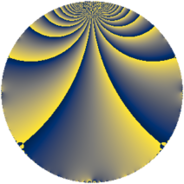# Properties

 Label 43.2.gLevel $43$ Weight $2$ Character orbit 43.g Rep. character $\chi_{43}(9,\cdot)$ Character field $\Q(\zeta_{21})$ Dimension $36$ Newform subspaces $1$ Sturm bound $7$ Trace bound $0$

# Related objects

## Defining parameters

 Level: $$N$$ $$=$$ $$43$$ Weight: $$k$$ $$=$$ $$2$$ Character orbit: $$[\chi]$$ $$=$$ 43.g (of order $$21$$ and degree $$12$$) Character conductor: $$\operatorname{cond}(\chi)$$ $$=$$ $$43$$ Character field: $$\Q(\zeta_{21})$$ Newform subspaces: $$1$$ Sturm bound: $$7$$ Trace bound: $$0$$

## Dimensions

The following table gives the dimensions of various subspaces of $$M_{2}(43, [\chi])$$.

Total New Old
Modular forms 60 60 0
Cusp forms 36 36 0
Eisenstein series 24 24 0

## Trace form

 $$36q - 10q^{2} - 16q^{3} - 18q^{4} - 17q^{5} - 4q^{6} + 6q^{7} + 18q^{8} - q^{9} + O(q^{10})$$ $$36q - 10q^{2} - 16q^{3} - 18q^{4} - 17q^{5} - 4q^{6} + 6q^{7} + 18q^{8} - q^{9} - 7q^{10} - 4q^{11} + 2q^{12} + 18q^{14} - 3q^{15} - 10q^{16} - 10q^{17} + 11q^{18} + 10q^{19} - 3q^{20} - 21q^{21} - 3q^{22} + 4q^{23} + 31q^{24} - 2q^{25} - 15q^{26} - 4q^{27} + 20q^{28} + 9q^{29} + 88q^{30} + 40q^{31} + 48q^{32} - 11q^{33} - 42q^{34} + 11q^{35} - 47q^{36} - 19q^{37} - 21q^{38} - q^{39} - 97q^{40} - 28q^{41} + 2q^{42} - 8q^{43} + 14q^{44} - 46q^{45} - 61q^{46} - 30q^{47} - 97q^{48} + 6q^{49} - 3q^{50} + 57q^{51} - 8q^{52} - 24q^{53} + 6q^{54} + 14q^{55} + 39q^{56} + 52q^{57} + 64q^{58} - q^{59} + 111q^{60} - 14q^{61} + 33q^{62} + 47q^{63} + 48q^{64} + 38q^{65} + 79q^{66} + 66q^{67} + 66q^{68} - 7q^{69} + 47q^{70} - 33q^{71} + 26q^{72} + 29q^{73} - 40q^{74} - 55q^{75} - 39q^{76} - 27q^{77} - 126q^{78} - 17q^{79} + 8q^{80} + 38q^{81} - 54q^{82} - 23q^{83} - 155q^{84} - 56q^{85} - 45q^{86} - 86q^{87} - 17q^{88} - 19q^{89} - 127q^{90} - 13q^{91} - 18q^{92} - 30q^{93} + 44q^{94} + q^{95} - 36q^{96} - 31q^{97} - 5q^{98} - 31q^{99} + O(q^{100})$$

## Decomposition of $$S_{2}^{\mathrm{new}}(43, [\chi])$$ into newform subspaces

Label Dim. $$A$$ Field CM Traces $q$-expansion
$$a_2$$ $$a_3$$ $$a_5$$ $$a_7$$
43.2.g.a $$36$$ $$0.343$$ None $$-10$$ $$-16$$ $$-17$$ $$6$$